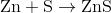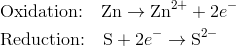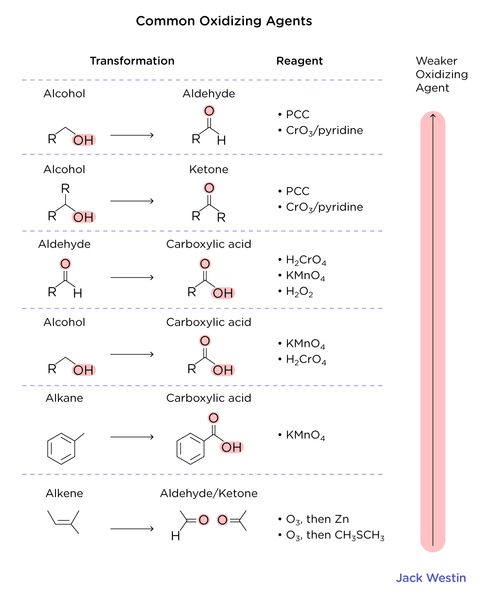Complimentary 1-hour tutoring consultation

MCAT Content / Stoichiometry / Oxidation Number

### Oxidation Number

Topic: Stoichiometry

The oxidation number is the effective charge on an atom in a compound; the oxidation number is useful in determining the nomenclature, or name, of chemical compounds.

The oxidation number describes explicitly the degree to which an element can be oxidized (lose electrons) or reduced(gain electrons). There is a fixed set of rules that can be followed to determine the oxidation number. The oxidation number can typically be found above groups in the periodic table.

1. The oxidation number of an atom is zero in a neutral substance that contains atoms of only one element. Thus, the atoms in O2, O3, P4, S8, and aluminium metal all have an oxidation number of 0.

2. The oxidation number of simple ions is equal to the charge on the ion. The oxidation number of sodium in the Na+ ion is +1, for example, and the oxidation number of chlorine in the Cl ion is -1.

3. The oxidation number of hydrogen is +1 when it is combined with a nonmetal as in CH4, NH3, H2O, and HCl.

4. The oxidation number of hydrogen is -1 when it is combined with metal as in LiH, NaH, CaH2, and LiAlH4.

5. The metals in Group IA form compounds (such as Li3N and Na2S) in which the metal atom has an oxidation number of +1.

6. The elements in Group IIA form compounds (such as Mg3N2 and CaCO3) in which the metal atom has a +2 oxidation number.

7. Oxygen usually has an oxidation number of -2. Exceptions include molecules and polyatomic ions that contain O-O bonds, such as O2, O3, H2O2, and the O22- ion.

8. The elements in Group VIIA often form compounds (such as AlF3, HCl, and ZnBr2) in which the nonmetal has a -1 oxidation number.

9. The sum of the oxidation numbers in a neutral compound is zero.

Redox reactions occur when one element is oxidized and another reduced in the same reaction. For example, the reaction between zinc and sulfur, which produces zinc sulfide:The half-reactions can be written:In the reaction above, the zinc is being oxidized by losing electrons. However, there must be another substance present that gains those electrons, and in this case, that is the sulfur. In other words, the sulfur is causing the zinc to be oxidized. Sulfur is called the oxidizing agent. The zinc causes the sulfur to gain electrons and become reduced and so the zinc is called the reducing agent. The oxidizing agent is a substance that causes oxidation by accepting electrons. The reducing agent is a substance that causes reduction by losing electrons. The simplest way to think of this is that the oxidizing agent is the substance that is reduced, while the reducing agent is the substance that is oxidized. The sample problem below shows how to analyze a redox reaction.A different type of reaction where redox can be seen is a disproportionation reaction, where a compound with an intermediate oxidation number converts into two compounds, one with a higher and one with a lower oxidation number. In the example of hydrogen peroxide decomposition oxygen is present in the reactant and both products. In H2O2, oxygen has an oxidation state of -1. In H2O, its oxidation state is -2, and it has been reduced. In Ohowever, its oxidation state is 0, and it has been oxidized. Oxygen has been both oxidized and reduced in the reaction.

2 H2O2(aq) → 2 H2O(l) + O2(g)

Practice Questions

Applications of Hard-Soft Acid-Base theory

MCAT Official Prep (AAMC)

Chemistry Question Pack Question 55

Chemistry Question Pack Passage 16 Question 89

Chemistry Question Pack Passage 19 Question 107

Chemistry Question Pack Passage 20 Question 115

Sample Test C/P Section Passage 5 Question 22

Practice Exam 2 C/P Section Passage 5 Question 24

Practice Exam 4 C/P Section Passage 1 Question 3

Key Points

• The oxidation number tells how many electrons the element can give or be given

• The oxidation state of a pure element is always zero.

• The oxidation state for a pure ion is equivalent to its ionic charge.

• In general, hydrogen has an oxidation state of +1, while oxygen has an oxidation state of -2.

• The sum of the oxidation states for all atoms of a neutral molecule must add up to zero.

• Reduction is the gain of electrons, which causes a decrease in oxidation state

• Oxidation is the loss of electrons, which causes an increase in oxidation state

• In disproportionate reactions, a chemical species is both reduced and oxidized at the same time

Key Terms

Oxidation: the loss of electrons in a substance (Cu to Cu+2)

Reduction: the gain of electrons in a substance (Cu+2 to Cu)

Disproportionation reaction: reaction where a compound with an intermediate oxidation number converts into two compounds, one with a higher and one with a lower oxidation number than the intermediate oxidation number

Oxidizing agent: a substance that causes oxidation by accepting electrons

Reducing agent: a substance that causes reduction by losing electrons

Oxidation number: the effective charge on an atom in a compound

Billing Information